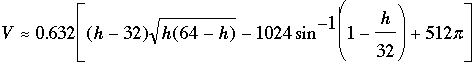SEARCH HOMEMath Central Quandaries & QueriesQuestion from Kinsley: Hello, I have a fuel tank with a broken gage. It is a 2000 gallon tank. THe dimensions are 64" dia. x 146" long. The fuel gage doesn't work, so the only measurement I can do is put a stick in it to see what is left. Currently it is at 19". PLEASE NOTE: the tank is laying on its side, meaning the long side is on the ground. So, how do I determine how much fuel is in the tank by the inches remaining? Thank you very much.Hello Kinsley,
Determining the level precisely requires some knowledge of Calculus.  Also, a couple assumptions are “built in” to the formula below:

1. The tank is perfectly level, and

2. The dipstick is perfectly plumb.

If we assume these conditions hold, then a (very good approximate) formula is given by:Where  h  is how many inches of fuel on the dipstick, and  V  is volume in U.S. gallons.

This formula is quite SCARY, and probably far more precise than necessary.

I recommend keeping a chart with the tank based on some handy divisions of the dipstick, such as this one:

 Dipstick Height (in.) 1 2 3 4 5 6 Etc.... Volume (US gal.) 6.7 18.9 34.5 52.9 73.6 96.2 Etc....

If you require better approximations divide these into smaller intervals, say of a half inch or perhaps quarter inch.

With such a large tank, small differences on the dipstick can make quite a difference when it comes to volume.

Write back if you need some clarification, or if you are interested in how the formula was obtained.

TylerMath Central is supported by the University of Regina and The Pacific Institute for the Mathematical Sciences.